# PHY105 Physics Lab Report Paper Editing and Proof Reading Services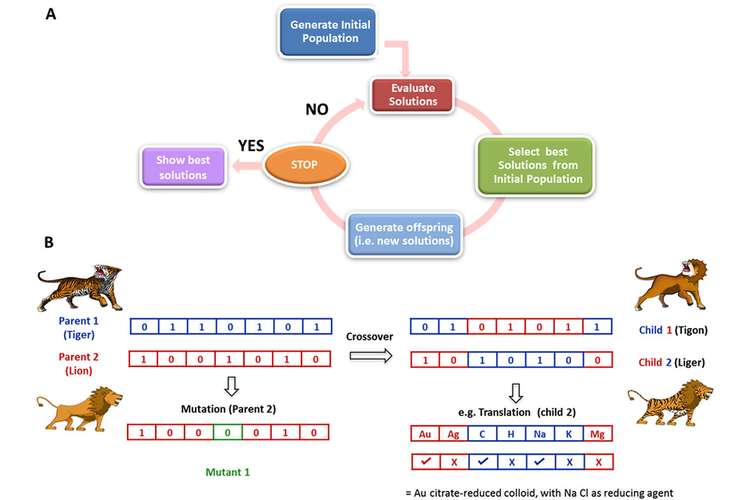## Introduction

In 1994, Broglie suggested that apart from familiar particle properties, these particles may have additional wave properties. He said that the particle’s wavelength is inversely proportional to its momentum as in equation (i) below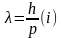Where p is momentum, h is Planck’s constant and λ wavelength of the particle. Currentry, experiments shows wave characters of electrons are proved by diffraction at a polycrystalline graphite lattice. Germer and Davisson experiment contradicts in that where electrons diffraction are observed in reflection where the setup uses transmission diffraction types like the one used by Thomson in the year 1928[ CITATION Lab \l 1033 ].

From equation (i), it is found that the momentum, p can be given as: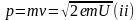Where m and v is the mass and velocity of the particle respectively, e is the electron charge and U is the accelerating voltage[ CITATION Lab \l 1033 ]. Thus,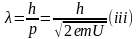According to L. Bragg, on the other hand, the wavelength of a particle is given by:[ CITATION Lab \l 1033 ]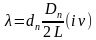Where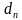is lattice plane spacing, L is distance between screen graphite and screen and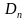is the ring diameter.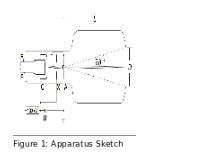### Apparatus

1. 1 Electron diffraction tube
2. 1 Tube stand
3. 1 High-voltage power supply 10 kV
4. Precision vernier callipers
5. Safety Connection Lead 25 cm red
6. Safety Connection Lead 50 cm red
7. 1 Safety Connection Lead 100 cm red
8. Safety Connection Lead 100 cm blue
9. Safety Connection Lead 100 cm black

### Procedure

1. Connected the cathode heating sockets F1 and F2 of the tube stand to the output on the back of the high-voltage power supply 10 kV
2. Connected the sockets C (cathode cap) and X (focussing electrode) of the tube stand to the negative pole
3. Connected the socket A (anode) to the positive pole of the 5 kV/2 mA output of the high-voltage power supply 10 kV
4. Grounded the positive pole on the high-voltage power electronics supply 10 kV
5. Applied an accelerating voltage U of 5 kV to observe the diffraction pattern.
6. The electron beam was focused relatively well, thus no magnet was required to alter the stream for more focused rings.
7. The accelerating voltage U was changed to 3 kV
8. The diameter of D1 (smaller ring) and D2 (larger ring) of the diffraction rings on the screen were measured
9. The voltage was increased to by 0.5kV and the rings measured again
10. Step 9 was repeated until a maximum of 5kV was reached.
11. Measured the distance between the graphite foil and the screen (L).
12. The wavelength of the light was determined by using Eq. 1 and the measured ring diameters. This was compared with the theoretical values (Eq. 2)
13. The ring diameters (D1 and D2) were plotted versus the inverse square root of U.
14. The slopes (k1 and k2) were determined by linear fits through the origin.
15. These values were compared to the given values for the lattice plane spacing

### Results

 U (kV) D1(cm) D2(cm) 3.0 2.90 5.20 3.5 2.70 4.50 4.0 2.40 4.36 4.5 2.20 4.00 5.0 2.07 3.81

Table 1: Diffraction Ring Diameters

 U (kV) D1(cm) ?1(pm) ?theory(pm) 3.0 2.90 23.50 22.4 3.5 2.70 21.88 20.7 4.0 2.40 19.45 19.4 4.5 2.20 17.83 18.3 5.0 2.07 16.78 17.3

Table 2: Calculated and Theoretical Wavelengths from Diffraction Ring 1

 U (kV) D2(cm) ?2(pm) ?theory(pm) 3.0 5.20 24.34 22.4 3.5 4.50 21.06 20.7 4.0 4.36 20.41 19.4 4.5 4.00 18.72 18.3 5.0 3.81 17.83 17.3

Table 3: Calculated and Theoretical Wavelengths from Diffraction Ring 1

The distance from screen to graphite foil, L is 13.14cm = 0.1314m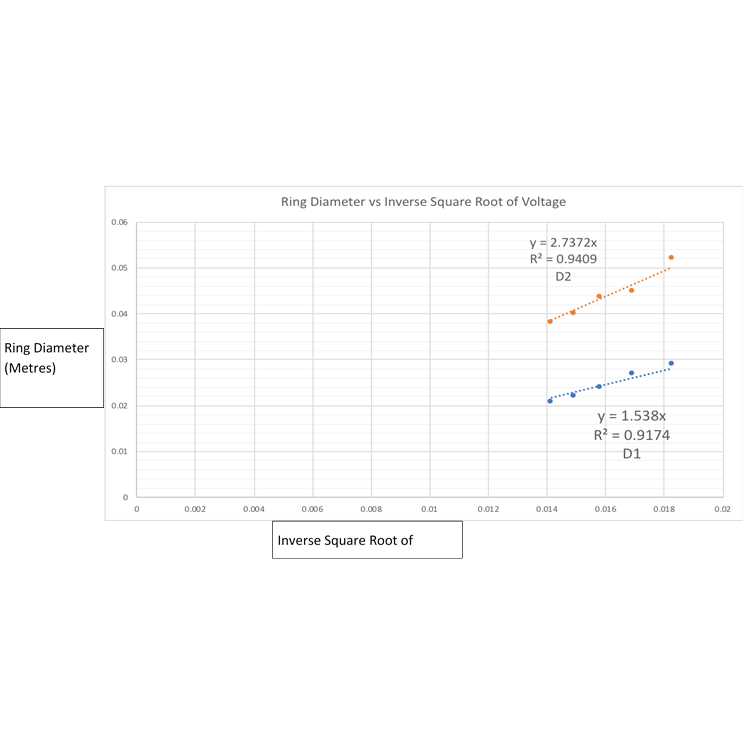Figure 2: The ring diameter vs. inverse square root ofvoltage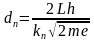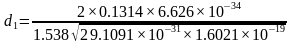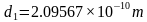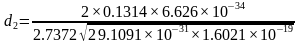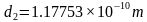### Discussion

In this lab, we have management supported particle-wave duality of the electron indirectly. As seen from table 1, we measured the inner and the outer diameters. In table 2, the wavelength was determined using the inner diameter and then compared to the theoretical values.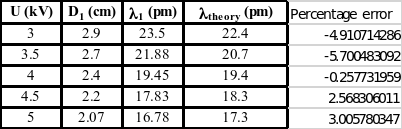In the calculation of percentage error, is very small which may arise due to human errors, errors due to fault/ or calibration error and any other errors that may arise when doing the experiment. The highest magnitude of percentage error is 5.7005 % which is still minimal.

Using the outer diameter, the wavelength of the particles were also done. Similarly there is small errors as compared to the theory values. The maximum magnitude percentage error in this part is 8.6607 which may be as a result of human and any other eroors.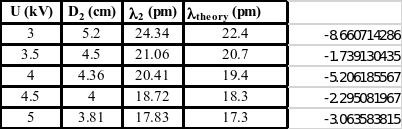Thus the DeBroglie relation was verified, though few errors making to have a slight different between the theoretical value and the experimental value.

#### Conclusions

In the psychology experiment, we were able to show that the wavelength follows the DeBroglie relation suggested in 1924. Finding the wavelengths of particles was also done, though the measured value was much closer to the accepted values. The per cent errors of -8.6607% to 3.0058% is comparatively small which may have caused by human error, apparatus calibration errors and/or any error may arise such as the temperature of the surrounding.

The final part was to calculate lattice plane spacing’s of graphite which was done successfully, though slight error as compared to the known values.

#### References

1. Lab Manual: Experiment 5: Dualism of Wave and Particles Electron Diffraction.